Test: Runtime Environment

# Test: Runtime Environment - Computer Science Engineering (CSE)

Test Description

## 20 Questions MCQ Test GATE Computer Science Engineering(CSE) 2023 Mock Test Series - Test: Runtime Environment

Test: Runtime Environment for Computer Science Engineering (CSE) 2023 is part of GATE Computer Science Engineering(CSE) 2023 Mock Test Series preparation. The Test: Runtime Environment questions and answers have been prepared according to the Computer Science Engineering (CSE) exam syllabus.The Test: Runtime Environment MCQs are made for Computer Science Engineering (CSE) 2023 Exam. Find important definitions, questions, notes, meanings, examples, exercises, MCQs and online tests for Test: Runtime Environment below.
Solutions of Test: Runtime Environment questions in English are available as part of our GATE Computer Science Engineering(CSE) 2023 Mock Test Series for Computer Science Engineering (CSE) & Test: Runtime Environment solutions in Hindi for GATE Computer Science Engineering(CSE) 2023 Mock Test Series course. Download more important topics, notes, lectures and mock test series for Computer Science Engineering (CSE) Exam by signing up for free. Attempt Test: Runtime Environment | 20 questions in 60 minutes | Mock test for Computer Science Engineering (CSE) preparation | Free important questions MCQ to study GATE Computer Science Engineering(CSE) 2023 Mock Test Series for Computer Science Engineering (CSE) Exam | Download free PDF with solutions
 1 Crore+ students have signed up on EduRev. Have you?
Test: Runtime Environment - Question 1

###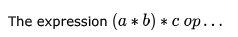where ‘op’ is one of ‘+’, ‘*’ and ‘ ’ (exponentiation) can be evaluated on a CPU with single register without  storing the value of (a * b) if

Detailed Solution for Test: Runtime Environment - Question 1

↑ has higer precedence than {*,+,-,/}

So, if op = ↑ implies, we need to evaluate the right hand side of ↑ first and then do the lhs part, which would definately require us to store the value of lhs

but if its a '+' or '*' , we dont need to store the values evaluated, and on the go can do the operation directly on one register.

Test: Runtime Environment - Question 2

### The program below uses six temporary variables a,b,c,d,e,f.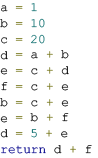Assuming that all operations take their operands from registers, what is the minimum number of registers needed to execute this program without spilling?

Detailed Solution for Test: Runtime Environment - Question 2

Here in these types of compiler questions, idea is "map/assign multiple temporaries to one registers."

here a, b, and c all are having 3 different values so i need atleast 3 registers r1, r2 and r3. a is mapped to r1, b to r2 and c to r3

d = a + b, after this line if u notice 'a' is never present on right hand side, so i can map (register of a which is r1 ) d to r1. e = c + d, after this line 'd' is never present on rhs, so i can map (register of d which is r1 ) e to r1.

at this time mapping is

r1 --- e

r2 --- b

r3 --- c

(at this moment i have registers for e, b and c. if i introduce new variable then i may need different register) now at this point if u see

f = c + e

b = c + e

these two are essentially doing same thing, after these two line 'b' and 'f' are same so i can skip computing 'f'. and whereever f is present i will replace it with 'b'. (bcoz neither of 'f' and 'b' are changing after these two lines, so value of these will be 'c+e' forever)

(seems like i introduced one more variable f, and register is needed for that, but actually i did not really introduce 'f'. i am skipping computation of 'f') now at second last line "d = 5 + e" here i introduced 'd', i can map it to any of the register r1 or r3, bcoz after this line neither of 'e' or 'c' is required. (value of 'b' is required bcoz i need to return 'd+f', and 'f' is essentially equal to 'b')

finally code becomes

r1 = 1

r2 = 10

r3 = 20

r1 = r1 + r2

r1 = r3 + r1

(skipping 'f' computation)

r2 = r3 + r1

r2 = r3 + r1

r1 = r2 + r2

r3 = 5 + r1

return r3 + r2

Therefore minimum 3 registers needed.

Test: Runtime Environment - Question 3

### Consider evaluating the following expression tree on a machine with load-store architecture in which memory can be accessed only through load and store instructions. The variables  a,b,c,d and e are initially stored in memory. The binary operators used in this expression tree can be evaluated by the machine only when operands are in registers. The instructions produce result only in a register. If no intermediate results can be stored in memory, what is the minimum number of registers needed to evaluate this expression?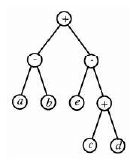Detailed Solution for Test: Runtime Environment - Question 3

Given is Load Store Architecture, that means we can access memory using Load and Store Instructions.

Key Idea:- Pick new register only when it is required.

We want to add c and d, and initially both are in memory, therefore copy these into registers.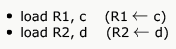(here no compensation can be done, we need two registers)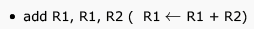(at this point R1 is holding c+d and R2 is holding d, i.e.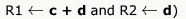Now, e comes into picture and my question is, Can i make use of R1 or R2 to store e ?
I can not use R1 to store e as its value will be needed later but I can use R2.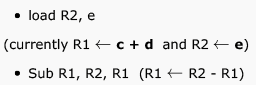Doing this all gives, final value of right sub-tree is stored in R1, and R2 stores e.
Now, coming to left subtree, to perform "a-b" we need to copy both variables in registers.
We can copy one of the variable in R2, but we can not obviously copy in R1 as value of R1 will be required later.

Load R3, b (here comes extra register, and we can not avoid using it.)

Current mapping is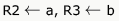and R1 contains final value of Right subtree.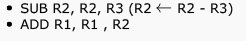Hence 3

*Answer can only contain numeric values
Test: Runtime Environment - Question 4

Consider the expression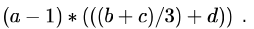Let X be the minimum number of registers required by an optimal code generation (without any register spill) algorithm for a load/store architecture, in which (i) only load and store instructions can have memory operands a n d (ii) arithmetic instructions can have only register or immediate operands. The value of X is _____________ .

Detailed Solution for Test: Runtime Environment - Question 4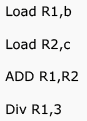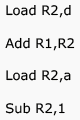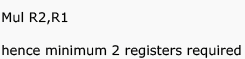Test: Runtime Environment - Question 5

In programming languages like C, C++, Python . . . the memory used by a program is typically separated into two parts, the stack and the heap.

Consider the following statements:

1. A stack is efficient for managing nested function calls.

2. Stack space is limited while heap space is not.

3. The stack cannot be used for persistent data structures.

Detailed Solution for Test: Runtime Environment - Question 5

Because Size of heap and stack both are limited. There is nothing which is unlimited. Yes but Size of stack and Size of heap is constant. It means that If there is total size available is 10, and If i used 7 block for stack then i can only use 3 block for heap but not more than that. i.e Size of stack and size of heap are inversely proportional. I mean that if size of stack increases then size of heap decreases, and vice versa.

Test: Runtime Environment - Question 6

A part of the system software which under all circumstances must reside in the main memory is:

Detailed Solution for Test: Runtime Environment - Question 6

The loader is a program that loads the object program from the secondary memory into the main memory for execution of the program. The loader resides in main memory.

Test: Runtime Environment - Question 7

A linker is given object modules for a set of programs that were compiled separately. What information need to be included in an object module?

Detailed Solution for Test: Runtime Environment - Question 7

(c) is the answer. For linker to link external symbols (for example in C, to link an extern variable in one module to a global variable in another module), it must know the location of all external symbols. In C external symbols includes all global variables and function names.
(a) is trivially there is an object module. (b) must be there if we need to have relocation capability.

Test: Runtime Environment - Question 8

A language L   allows declaration of arrays whose sizes are not known during compilation. It is required to make efficient use of memory. Which one of the following is true?

Detailed Solution for Test: Runtime Environment - Question 8

If a language L allows declaration of arrays whose sizes are not known during compilation time. It is required to use efficient use of memory.
So a compiler using dynamic memory allocation can be written for L.

An array is a collection of data items, all of the same type, accessed using a common name.

C dynamic memory allocation refers to performing manual memory management for dynamic memory allocation in the C programming language via a group of functions in the C standard library, namely malloc, realloc, calloc and free.

Test: Runtime Environment - Question 9

In a resident – OS computer, which of the following systems must reside in the main memory under all situations?

Detailed Solution for Test: Runtime Environment - Question 9

In many operating systems the loader is permanently resident in memory, although some operating systems that support virtual memory may allow the loader to be located in a region of memory that is pageable.

Test: Runtime Environment - Question 10

Faster access to non-local variables is achieved using an array of pointers to activation records called a

Detailed Solution for Test: Runtime Environment - Question 10

properties of displays

1>    Use a pointer array to store the activation records along the static chain.

2>    Fast access for non-local but may be complicated to maintain.

3>    Calling a subprogram in the same level – simply replace and restore.

4>    Calling a subprogram in the higher level – add an entry and may need to save the old pointers.

5>    Calling a subprogram in the lower level – shrink the pointer and restore it when the subprogram returns.

Test: Runtime Environment - Question 11

A linker reads four modules whose lengths are 200,800,600,and 500 words, respectively. If they are loaded in that order, what are the relocation constants?

Detailed Solution for Test: Runtime Environment - Question 11

first module loaded starting at address 0. Size is 200. hence it will occup first 200 address last address being 199. Second module will be present from 200 and so on.

Test: Runtime Environment - Question 12

The process of assigning load addresses to the various parts of the program and adjusting the code and the data in the program to reflect the assigned addresses is called

Detailed Solution for Test: Runtime Environment - Question 12

Relocation is the process of assigning load addresses to position-dependent code of a program and adjusting the code and data in the program to reflect the assigned addresses.

Hence Option C is Ans
Symbol resolution  is the process of searching files and libraries to replace symbolic references or names of libraries with actual usable addresses in memory before running a program.

Test: Runtime Environment - Question 13

Which one of the following is NOT performed during compilation?

Detailed Solution for Test: Runtime Environment - Question 13

Dynamic means- at runtime. Dynamic memory allocation happens during the execution time and hence (A) is the answer.

Test: Runtime Environment - Question 14

Consider the following intermediate program in three address code

p = a - b

q = p * c

p = u * v

q = p + q

Which one of the following corresponds to a static single assignment form of the above code?

Detailed Solution for Test: Runtime Environment - Question 14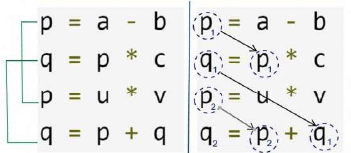Test: Runtime Environment - Question 15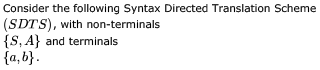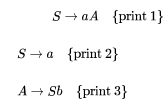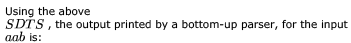Detailed Solution for Test: Runtime Environment - Question 15

aab could be derived as follows by the bottom up parser:

S->aA prints 1

A->aSb prints 3

A->aab  prints 2

Now since bottom up parser will work in reverse of right most derivation, so it will print in bottom up fashion i.e., 231 which is option C.

Note that this could also be visualized easily by drawing the derivation tree.

Test: Runtime Environment - Question 16

A shift reduce parser carries out the actions specified within braces immediately after reducing with the corresponding rule of grammar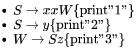What is the translation of xxxxyzz using the syntax directed translation scheme described by the above rules?

Detailed Solution for Test: Runtime Environment - Question 16

Making a tree and performing post order traversal will yield answer as A.

S-->x x W (Pf'1')

W-->S z (Pf'3')

S-->x x W (Pf'1')

W-->S z (Pf'3')

S-->y (Pf'2').

Test: Runtime Environment - Question 17

In a bottom-up evaluation of a syntax directed definition, inherited attributes can

Detailed Solution for Test: Runtime Environment - Question 17

A Syntax Directed Definition (SDD) is called S Attributed if it has only synthesized attributes.

L-Attributed Definitions contain both synthesized and inherited attributes but do not need to build a dependency graph to evaluate them.

Test: Runtime Environment - Question 18

Which one of the following is TRUE at any valid state in shift-reduce parsing?

Detailed Solution for Test: Runtime Environment - Question 18

A handle is actually the one which is always on the top of the stack. A viable prefix(prefix of the Right-hand side of a production or productions), is actually a prefix of the handle and so can never extend past the right end of the handle(i.e. the top of the stack).
The structure of the stack can be considered as a set of viable prefixes -

Test: Runtime Environment - Question 19

Consider the grammar rule E → E1 – E2 for arithmetic expressions. The code generated is targeted to a CPU having a single user register. The subtraction operation requires the first operand to be in the register. If E1 and E2 do not have any common sub expression, in order to get the shortest possible code

Detailed Solution for Test: Runtime Environment - Question 19

E2 should be evaluated first

After evaluating E2 first and then E1, we will have E1 in the register and thus we can simply do SUB operation with E2 which will be in memory (as we have only a single register). If we do E1 first and then E2, we must move E2 to memory and E1 back to register before doing SUB, which will increase the code size.

*Answer can only contain numeric values
Test: Runtime Environment - Question 20

Consider the following code segment.

x = u - t;

y = x * v;

x = y + w;

y = t - z;

y = x * y;

The minimum number of total variables required to convert the above code segment to static single assignment form is __________.

Detailed Solution for Test: Runtime Environment - Question 20

x(temp3) = u(temp1) - t(temp2);

y(temp5) = x * v(temp4);

x(temp7) = y + w(temp6);

y(temp9) = t - z(temp8);

y(temp10) = x * y;

## GATE Computer Science Engineering(CSE) 2023 Mock Test Series

151 docs|215 tests
Information about Test: Runtime Environment Page
In this test you can find the Exam questions for Test: Runtime Environment solved & explained in the simplest way possible. Besides giving Questions and answers for Test: Runtime Environment, EduRev gives you an ample number of Online tests for practice

## GATE Computer Science Engineering(CSE) 2023 Mock Test Series

151 docs|215 tests(Scan QR code)